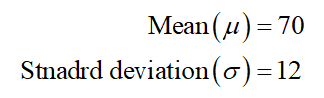# A normal distribution has a mean of µ = 70 with σ = 12. If one score is randomly selected from this distribution, what is the probability that the score will be greater than X = 55

Question
18 views

A normal distribution has a mean of µ = 70 with σ = 12. If one score is randomly selected from this distribution, what is the probability that the score will be greater than X = 55

check_circle

Step 1

According to the provided information,...

### Want to see the full answer?

See Solution

#### Want to see this answer and more?

Solutions are written by subject experts who are available 24/7. Questions are typically answered within 1 hour.*

See Solution
*Response times may vary by subject and question.
Tagged in